# Python Program to Convert Decimal to Binary

In this article, we've created some programs in Python to convert decimal number to its equivalent binary value. The decimal number must be entered by user at run-time. Here are the list of programs:

• Decimal to Binary using list
• Without using list
• Using function
• Using class
• Using bin()
• Shortest Python Code for Decimal to Binary Conversion

Note - Before creating these programs, if you're not aware about steps used for the conversion, then refer to Decimal to Binary Formula and Steps to get every required things about the topic.

## Decimal to Binary using List

To convert decimal to binary number in Python, you have to ask from user to enter a number in decimal number system, then convert that number into its binary equivalent as shown in the program given below:

```print("Enter the Decimal Number: ")
dnum = int(input())
i = 0
bnum = []
while dnum!=0:
rem = dnum%2
bnum.insert(i, rem)
i = i+1
dnum = int(dnum/2)

i = i-1
print("\nEquivalent Binary Value is:")
while i>=0:
print(end=str(bnum[i]))
i = i-1
print()```

Here is its sample run: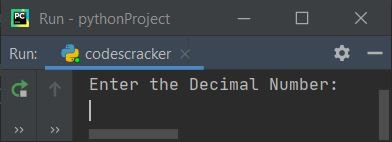Now enter any number in decimal number system say 50 and then press `ENTER` key to convert and print its equivalent value in binary number system as shown in the snapshot given below:The dry run of above program with user input 50 goes like:

• Initial values, dnum=50 (entered by user), i=0
• Now the condition (of while loop) dnum!=0 or 50!=0 evaluates to be true, therefore program flow goes inside the loop
• dnum%2 or 50%2 or 0 gets initialized to rem
• Using the following statement
`bnum.insert(i, rem)`
the value of rem gets initialized to bnum[i]. Therefore rem or 0 gets initialized to bnum[i] or bnum
• i+1 or 0+1 or 1 gets initialized to i
• int(dnum/2) or int(50/2) or 25 gets initialized as new value of dnum
• Now program flow goes back and evaluates the condition of while loop again. That is, the condition dnum!=0 or 25!=0 evaluates to be true again, therefore program flow again goes inside the loop and evaluates all the four statements of its body
• This process continues, until the condition evaluates to be false
• Before condition evaluates to be false, here are the list of values one by one after each evaluation of the loop:
• At first execution:
• rem = 0
• bnum = 0
• i = 1
• dnum = 25
• At second execution:
• rem = 1
• bnum = 1
• i = 2
• dnum = 12
• At third execution:
• rem = 0
• bnum = 0
• i = 3
• dnum = 6
• At fourth execution:
• rem = 0
• bnum = 0
• i = 4
• dnum = 3
• At fifth execution:
• rem = 1
• bnum = 1
• i = 5
• dnum = 1
• At sixth execution:
• rem = 1
• bnum = 1
• i = 6
• dnum = 0
• In this way, the list bnum[] has its value as:
• bnum = 0
• bnum = 1
• bnum = 0
• bnum = 0
• bnum = 1
• bnum = 1
• Now print the value of bnum[] list from its last to 0th index.
• Therefore, the binary equivalent of given decimal number (50) is 110010

#### Modified Version of Previous Program

This is the modified version of previous program. The end= is used to skip printing of an automatic newline using print(). The str() method is used to convert any type of value to a string type.

```print("Enter Decimal Number: ", end="")
d = int(input())
i = 0
b = []
while d!=0:
b.insert(i, d % 2)
i = i+1
d = int(d / 2)

i = i-1
print(end="\nEquivalent Binary Value = ")
while i>=0:
print(end=str(b[i]))
i = i-1
print()```

Here is its sample run with user input, 105 as decimal number:## Decimal to Binary without List

This program does the same job as of previous program, but without using list. You can take its dry run with yourself to understand it in better way:

```print("Enter Decimal Number: ", end="")
dnum = int(input())

bnum = 0
mul = 1
while dnum>0:
rem = dnum%2
bnum = bnum+(rem*mul)
mul = mul*10
dnum = int(dnum/2)

print("\nEquivalent Binary Value =", bnum)```

Here is its sample run with user input, 345 as decimal number input: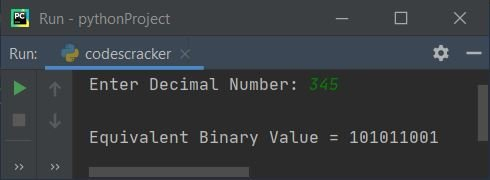## Decimal to Binary using Function

This program uses a user-defined function named DecToBin() that receives a number as its argument and returns its equivalent binary value. Therefore, we've passed the decimal number entered by user to this function, so that its return value gets initialized to bnum. And the value of this variable gets printed on output as binary equivalent of given decimal number by user at run-time:

```def DecToBin(d):
b = 0
m = 1
while d>0:
b = b + ((d%2)*m)
m = m*10
d = int(d/2)
return b

print("Enter Decimal Number: ", end="")
dnum = int(input())

bnum = DecToBin(dnum)
print("\nEquivalent Binary Value =", bnum)```

This program produces same output as of previous program.

## Decimal to Binary using Class

This is the last program, created using a class named CodesCracker. Class is an object-oriented feature of Python. To access anything like member function of the class, we've to create an object before doing this

Therefore an object named ob is created of class CodesCracker. So that, we can access its member function named DecToBin() using dot (.) operator to do the job. Rest of the things works similar to a normal function.

```class CodesCracker:
def DecToBin(self, d):
b = 0
m = 1
while d>0:
b = b + ((d%2)*m)
m = m*10
d = int(d/2)
return b

print("Enter Decimal Number: ", end="")
dnum = int(input())

ob = CodesCracker()
bnum = ob.DecToBin(dnum)
print("\nEquivalent Binary Value =", bnum)```

## Decimal to Binary using bin()

This is the program that uses bin(), a predefined function of Python to do the same job as previous programs have done. The bin() function returns binary equivalent of value passed as its argument.

```print("Enter Decimal Number: ", end="")
dnum = int(input())

bnum = bin(dnum)
print("\nEquivalent Binary Value =", bnum)```

Here is its sample run with user input, 49: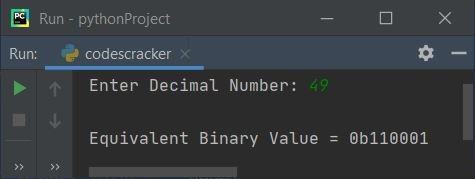Note - To remove first two characters, add [2:] just after bnum while printing its value to print all its element starting from second index. Or just replace the following statement:

`print("\nEquivalent Binary Value =", bnum)`

with the statement given below:

`print("\nEquivalent Binary Value =", bnum[2:])`

now the output with same user input, that is 49 looks like: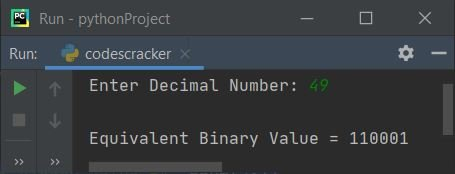### Shortest Python Code for Decimal to Binary Conversion

This is the shortest Python code for decimal to binary conversion. This program doesn't print any message. The program receives input from user, and print its binary equivalent, without any extra things like previous programs have:

```dnum = int(input())
print(bin(dnum)[2:])```

Here is its sample run with user input 59: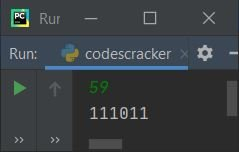#### Same Program in Other Languages

Python Online Test

« Previous Program Next Program »